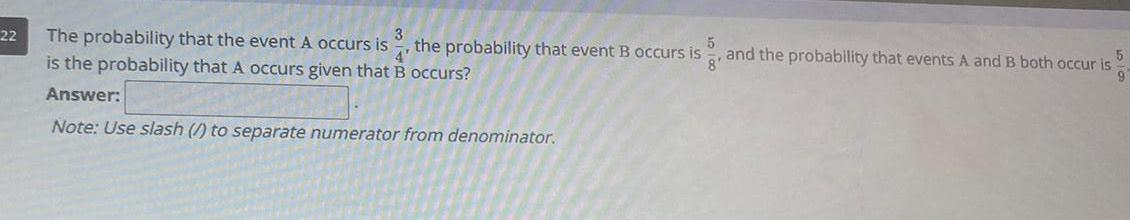Question:

# 22 The probability that the event A occurs is 3 is the

Last updated: 9/7/202322 The probability that the event A occurs is 3 is the probability that A occurs given that B occurs Answer Note Use slash to separate numerator from denominator the probability that event B occurs is 5 8 and the probability that events A and B both occur is 9